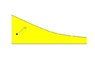# Analysis Question

amcavoy
This is a problem I found on the internet. In fact, I'm not even in analysis but I'm interested in how it's done.

Consider a smooth function $f:(0,\infty)\rightarrow\mathbb{R}$ such that $f(x)>0$ and $\lim_{x\to\infty}f(x)=0$. f(x) is convex and decreasing. Consider now a region between the positive x-axis and f(x). Think of it as a room and the positive real axis and f(x) as the walls. Now take a ball and throw it inside the room. When it touches the wall, it reflects by the usual law. Prove that after some time the ball will come back and exit the room.

#### Attachments

•billiard.jpg
5.1 KB · Views: 404